## Samacheer Kalvi 3rd Standard Maths Book Solutions Term 1 Chapter 6 Information Processing

Activity 1 (Text Book Page No. 57):

Let us colour the blocks.

Find all possible ways of colouring the given blocks with blue and red. One is done for you.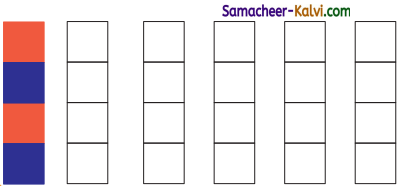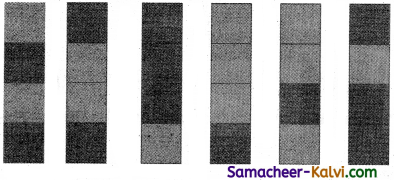Activity 2 (Text Book Page No. 57):

Fill the white space in the given blocks with alternate colours and answer the following questions based on it.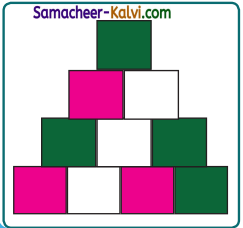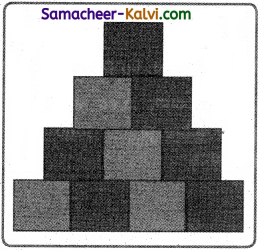Question 1.
Total number of blocks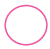10

Question 2.
Number of rose block4Question 3.
Number of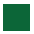blocks6

Question 4.
Number of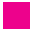blocks in 2 and 4 row1 & 2

Question 5.
How manymorethan2Practice (Text Book Page No. 58):
Question 3.
List down all possible ways of forming three digit numbers by using the given digits only once.

a. 9, 6, 8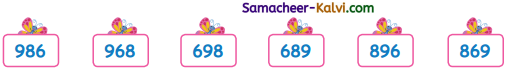b. 3, 2, 0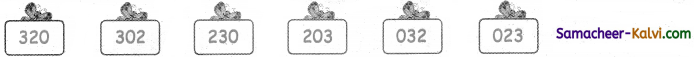c. 1, 5, 4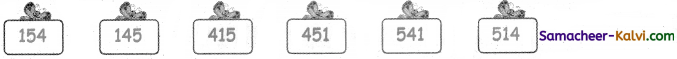Question 4.
Find all possible ways of combining the three letters a, e, tusing each of them once.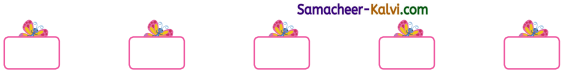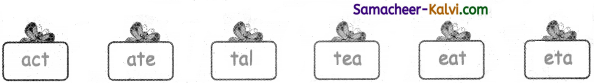Practice (Text Book Page No. 60):

Question 1.
Collect the data from 40 of your friends about their favourite food and represent it in Picture.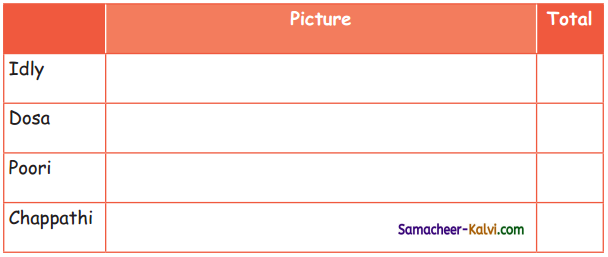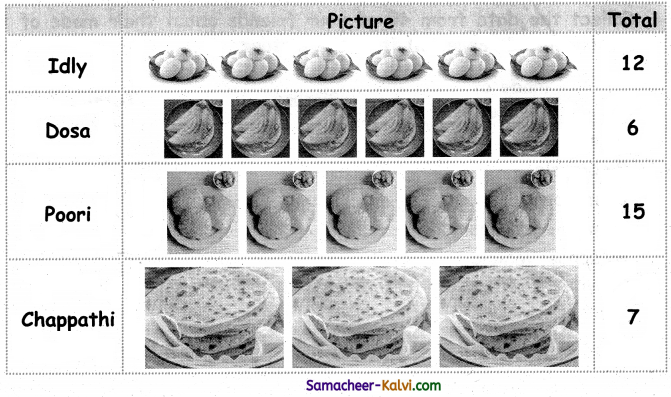Question 2.
The following picture represents the number of chocolates sold at a shop in a week.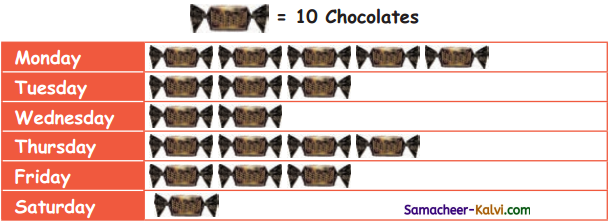Answer the following questions from the data given in the above table.

Question 1.
The total number of chocolates sold on Thursday is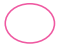40

Question 2.
The sale was maximum on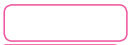MondayQuestion 3.
The sale was minimum onSaturday

Question 4.
Sale were equal onandTuesday, Friday

Question 5.
The total number of Chocolates sold in six days are180Question 3.
Collect the data from 40 of your friends about their mode of travel to school and represent it in the picture.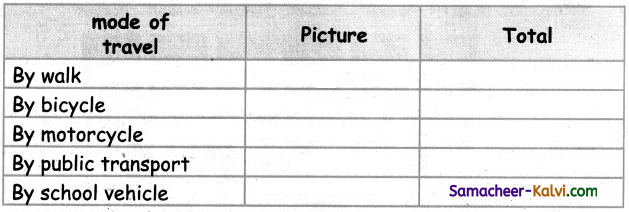Activity to be done by the students themselves.Draw a conclusion from the representation by discussing with your teacher.

The graph below shows the number of children in a school studying in classes 1-4 of a school. The number of girls studying in classes 1-4 of a school are 14 10, 16 and 13 respectively. braw the graph discussing with your teacher for number of boys studying in classes 1-4.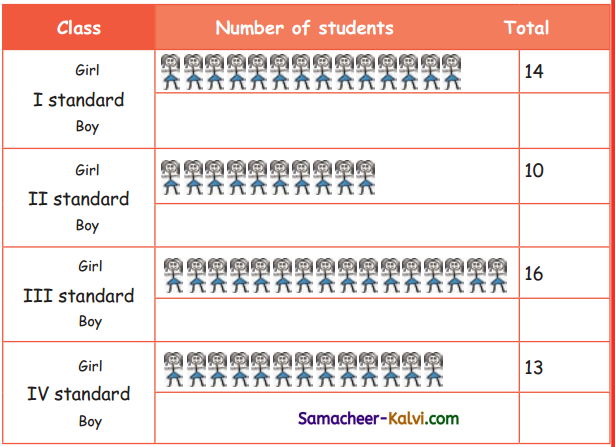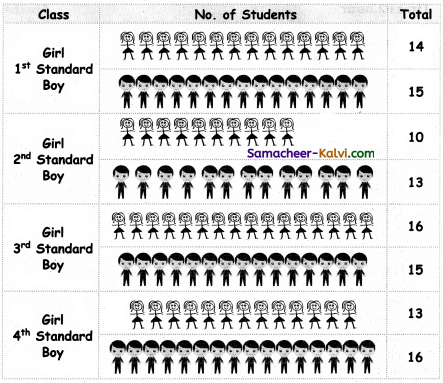After completing the pictorial representation answer the following questions.

i. The number of girls in class 2 is ___________.
10

ii. The number of boys in class 3 is ___________.
15

iii. The total number of students in class 4 is ___________.
29

iv. The total number of girls from class 1 to 4 is ___________.